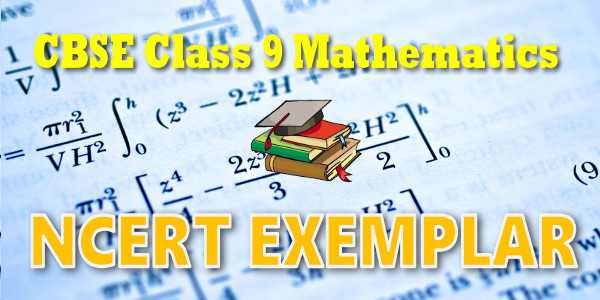No products in the cart.

# CBSE - Class 09 - Mathematics - Linear Equations in Two Variables - NCERT Exemplar Solutions

## NCERT Exemplar Solutions for Mathematics Linear Equations in Two Variables### Student Subscription

##### Unlock the exclusive content designed for the toppers

We will update content for this category shortly. Please visit this category after few days or subscribe to our newsletter by email for latest updates

# NCERT Exemplar Solutions for class 9 Mathematics Linear equation in two variables# NCERT 9 Mathematics Exemplar Problem Text book Solutions

## NCERT Exemplar Problems Solutions

• NCERT Exemplar Problems Solutions for Class 9 Mathematics PDF
• NCERT Exemplar Problems Class 9 Mathematics - CBSE
• NCERT Exemplar Problems-Solutions MATHEMATICS class 9th
• NCERT Exemplar Problems Solutions for Class 9 Mathematics
• NCERT Exemplar Problems class 9 Mathematics

## NCERT Class 09 Mathematics Chapter-wise Exemplar Solutions

• Chapter 01: Number System
• Chapter 02: Polynomials
• Chapter 03: Coordinate geometry
• Chapter 04: Linear equation in two variables
• Chapter 05: Introduction to Euclid’s geometry
• Chapter 06: Lines and angles
• Chapter 07: Triangles
• Chapter 09: Areas of parallelograms and triangles
• Chapter 10: Circles
• Chapter 11: Construction
• Chapter 12: Heron’s formula
• Chapter 13: Surface areas and volumes
• Chapter 14: Statistics
• Chapter 15: Probability

Here is the list of topics covered under each chapter of class 9 Mathematics NCERT text book.

## NCERT Exemplar Problem book and Solutions for Class 9th Mathematics

NCERT exemplar problem book and Solutions Class 9 Mathematics PDF (Download) Free from myCBSEguide app and myCBSEguide website. Ncert solution class 9 Mathematics includes text book solutions from both book. NCERT Solutions for CBSE Class 9 Mathematics have total 15 chapters. Class 9 Mathematics ncert exemplar problem and Solutions in pdf for free Download are given in this website. NCERT 9 Mathematics Exemplar Problem Text book Solutions.Ncert Mathematics class 9 exemplar solutions PDF and Mathematics ncert class 9 PDF exemplar problems and solutions with latest modifications and as per the latest CBSE syllabus are only available in myCBSEguide.

### CHAPTER FOUR LINEAR EQUATION IN TWO VARIABLE

• 4.1 Linear equations
• 4.2 Solution of linear equations
• 4.3 Graph of a linear equation in two variables
• 4.4 Equations of Lines Parallel to x-axis and y-axis

### NCERT Exemplar Problems & Solutions

NCERT exemplar text books are available in NCERT official website for free download. To download sample paper for class 9 Science, Social Science, Mathematics, English Communicative, English Language and Literature, Hindi Course-A, Hindi Course-B, Sanskrit, Foundation of IT, French, Painting, Home Science, Music; do check myCBSEguide app or website. myCBSEguide provides sample papers with solution, test papers for chapter-wise practice, NCERT solutions, NCERT Exemplar solutions, quick revision notes for ready reference, CBSE guess papers and CBSE important question papers. Sample Paper all are made available through the best app for CBSE students and myCBSEguide website.## myCBSEguide

Trusted by 1 Crore+ Students## Test Generator

Create papers online. It's FREE.## CUET Mock Tests

75,000+ questions to practice only on myCBSEguide app